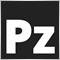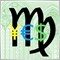# Question about POINT_VALUE/TICK_VALUE in CFDs (solved)720103

Hi everyone!

I hope you are fine. I have a dumb question. I have problems auto-calculating lotsizes for CFDS -only on some brokers-.

On *some* cfds, the tick value defined by the broker is wrong, or at least I think it is.

For example, for the symbol SP500 at GlobalPrime....

MarketInfo(Symbol(), MODE_POINT) is 0.01
MarketInfo(Symbol(), MODE_TICKVALUE) is 0.1

But once a trade is taken, each point of the price movement impacts the account by 10\$, not 0.1\$.

Is the TICK_VALUE wrong? Or I am doing something wrong and CFDs need a more particular code? The following is my lotsize calculation logic:

// Desired risk in money
double desired_risk_in_money = AccountEquity() * (DesiredRisk/100)); // DesiredRisk is INT parameter

// Current Stop
double sl = SL*GetDecimalPip(); // SL is INT parameter

// Tick value in account currency
double pointvalue = MarketInfo(Symbol(), MODE_TICKVALUE);

// Stoploss in basic points
double sl_in_points = sl/MarketInfo(Symbol(), MODE_POINT);

// Risk in money for one lote
double risk_in_money_for_1lot = sl_in_points * pointvalue;

double lots = desired_risk_in_money / risk_in_money_for_1lot; // Desired lots

The GetDecimalPip() function is...

double GetDecimalPip()
{
switch(Digits)
{
case 5: return(0.0001);
case 4: return(0.0001);
case 3: return(0.01);
case 2: return(0.1);
case 1: return(1);
default: return(1);
}
}

The above logic works fantastic for all forex pairs, including oil, gold and silver. Only cfds seem out of whack.
Thanks everyone!1031

I am having same problem with the E Mini S&P futures contract or ES in MT5. It moves in increments of a quarter point or 0.25 and 4 makes a point. So I had to use 2500 for a tick or 10000 for one whole point. Still trying to figure things out as I am not that good at code.

Thanks.1031

And in US Bonds one tick is \$31.25 and 32 ticks make a full point or \$1000. So it goes up to 99,999 to get the 32 ticks in a point. So a 3 tic stop or \$93.75 is input not as 3 but 9375. Makes sense? I am still learning too. Wish it was easier in MT5.

Thanks.23788

There is Tick, PIP, and Point. They are all different in general. A tick is the smallest change of price. A Point is the least significant digit quoted. In currencies a pip is defined as 0.0001 (or for JPY 0.01)

On a 4 digit broker a point (0.0001) = pip (0.0001). [JPY 0.01 == 0.01] On a 5 digit broker a point (0.00001) = 1/10 pip (0.00010/10). Just because you quote an extra digit doesn't change the value of a pip. (0.0001 == 0.00010) EA's must adjust pips to points (for mq4.) In currencies a tick is a point. Price can change by least significant digit (1.23456 -> 1.23457)

In metals a Tick is still the smallest change but is larger than a point. If price can change from 123.25 to 123.50, you have a TickSize of 0.25 and a point of 0.01. Pip has no meaning.

This is why you don't use TickValue by itself. Only as a ratio with TickSize. See DeltaValuePerLot()Moderator
26749

Arturo Lopez Perez:

But once a trade is taken, each point of the price movement impacts the account by 10\$, not 0.1\$.

The GetDecimalPip() function is...

double GetDecimalPip()
{
switch(Digits)
{
case 5: return(0.0001);
case 4: return(0.0001);
case 3: return(0.01);
case 2: return(0.1);
case 1: return(1);
default: return(1);
}
}

Why are you returning 0.1 for 2 Digits?

Tickvalue is supposed to be in the account currency, but with many brokers is is not for non forex symbols. For USA indices it may return USD and ie DAX, Euros.

What is your account currency and how are you determining that each point of the price movement impacts the account by 10\$, not 0.1\$ ?Moderator
26749

Is the TICK_VALUE wrong? Or I am doing something wrong and CFDs need a more particular code? The following is my lotsize calculation logic:

As a matter of interest, try this in a script

int ticket=INSERT TICKET # HERE;
if(OrderSelect(ticket,SELECT_BY_TICKET))
{
double tv=MarketInfo(OrderSymbol(), MODE_TICKVALUE);
double ts=MarketInfo(OrderSymbol(), MODE_TICKSIZE);
double MIpoint=MarketInfo(OrderSymbol(), MODE_POINT);
int dig=(int)MarketInfo(OrderSymbol(), MODE_DIGITS);
Print(OrderSymbol()," MarketInfo Point value is ",DoubleToStr(MIpoint,dig));
double profit=OrderProfit();
Print(OrderSymbol()," profit (currency) is ",DoubleToStr(profit,2));
double profitdecimal=0;
profitdecimal=OrderClosePrice()-OrderOpenPrice();
if(OrderType()==OP_SELL)
profitdecimal=OrderOpenPrice()-OrderClosePrice();
Print(OrderSymbol()," profit (decimal) is ",DoubleToStr(profitdecimal,dig));
int ticks=(int)MathRound(profitdecimal/ts);
Print(OrderSymbol()," profit as multiples of tick size is ",ticks);
double ppt=profit/ticks/OrderLots();
Print(OrderSymbol()," calculated TickValue is ",DoubleToStr(ppt,2));
Print(OrderSymbol()," MarketInfo TickValue is ",DoubleToStr(tv,2));
}

and see how they compare31868

Amount which every point change costs you depends from order lots. You did not show this in the codes.

Meanwhile try this:

double VolumeCalculateForRisk(string symbol, double risk, int StopLossPoints)
{
double margin = AccountFreeMargin();
double Loss = risk * margin;
double volume = Loss / (StopLossPoints * MarketInfo(symbol, MODE_POINT) * MarketInfo(symbol, MODE_TICKVALUE) / MarketInfo(symbol, MODE_TICKSIZE));
return(volume);
}23788

Arturo Lopez Perez: But once a trade is taken, each point of the price movement impacts the account by 10\$, not 0.1\$.

Wrong. If you trade the EURUSD with a USD account currency, then it is (exactly) \$10/pip/lot \$1/point/lot (5 digit broker).

1 pip * tickValue / tickSize = 0.0001 * 1 / 0.00001 = \$10/lot. Unless you know tick value and tick size you know nothing. If tick value/tick size is wrong, complain to your broker.

• You place the stop where it needs to be - where the reason for the trade is no longer valid. E.g. trading a support bounce the stop goes below the support.
• Account Balance * percent/100 = RISK = OrderLots * (|OrderOpenPrice - OrderStopLoss| * DeltaPerLot + CommissionPerLot) (Note OOP-OSL includes the SPREAD, and DeltaPerLot is usually around \$10/pip but it takes account of the exchange rates of the pair vs. your account currency.)
• Do NOT use TickValue by itself - DeltaPerLot
• You must normalize lots properly and check against min and max.
• You must also check FreeMargin to avoid stop out720103

Keith Watford:

Why are you returning 0.1 for 2 Digits?

In gold, for instance, a pip os 0.1. Two-digit symbols follow that pip value.Moderator
26749

Arturo Lopez Perez:
In gold, for instance, a pip os 0.1. Two-digit symbols follow that pip value.

Yes, but what would happen if a broker happened to only have 2 Digits for Japanese pairs?

Yes I know that it is probably very rare nowadays.

Did you put my code in a script and check the trade?40867

Arturo Lopez Perez:

Hi everyone!

I hope you are fine. I have a dumb question. I have problems auto-calculating lotsizes for CFDS -only on some brokers-.

On *some* cfds, the tick value defined by the broker is wrong, or at least I think it is.

For example, for the symbol SP500 at GlobalPrime....

MarketInfo(Symbol(), MODE_POINT) is 0.01
MarketInfo(Symbol(), MODE_TICKVALUE) is 0.1

But once a trade is taken, each point of the price movement impacts the account by 10\$, not 0.1\$.

Is the TICK_VALUE wrong? Or I am doing something wrong and CFDs need a more particular code? The following is my lotsize calculation logic:

There is obviously a wrong tick value. You should report it to GlobalPrime.

However you should avoid to mix point and tick. A point is not always the same as a tick, so you have to use ticksize instead of point (size).